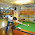Source: Flipkart Interview Question from Interview Street (taken from Chinmay - CSE IITB Blog)

Problem:

Find the smallest number of jumps (i.e. optimal number of dice throws) needed to win a snakes and ladders game. Assume you are given a board with all the necessary inputs like start/end positions of all ladders and snakes.

1.DAG, with each number as a node. A number x is linked to x + 1, x + 2, .. x + 6 with edge 1. A number with snake's head is linked only to the snakes tail with edge weight 0. A number with ladder's tail is linked only to the number with ladder's head with weight 0.
BFS with destination = final point.

Maximum number of edges from a vertex = 6. At worse, I travel each edge exactly once. So, at definitely it is O(n). Although, I am reasonably sure, the complexity in this case will come out to be much lesser.

1.Its not a DAG as graph is not acyclic!

2.this question also came during flipkart hiring process at other IITs , i also thought it was a DAG, but one can easily form a cycle here using a snake ,plus the edge weights were different so went with dijkstra later , (however wasn't able to code dijkstra during the time limit , so had to contend with BFS code which wasnt giving full score :/ )

3.I would say it seems like an application of Djiktra's algorithm with source as 0 and destination as 100. Considering every move as unit cost, we just have to find the shortest path... And everything needed is given as input hence we have a graph with edges and weights.